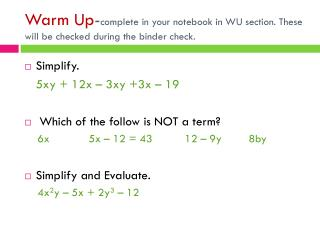DownloadDownload PresentationSimplify. 5xy + 12x – 3xy +3x – 19 Which of the follow is NOT a term?

# Simplify. 5xy + 12x – 3xy +3x – 19 Which of the follow is NOT a term?

Télécharger la présentation## Simplify. 5xy + 12x – 3xy +3x – 19 Which of the follow is NOT a term?

- - - - - - - - - - - - - - - - - - - - - - - - - - - E N D - - - - - - - - - - - - - - - - - - - - - - - - - - -
##### Presentation Transcript

1. Warm Up-complete in your notebook in WU section. These will be checked during the binder check. • Simplify. 5xy + 12x – 3xy +3x – 19 • Which of the follow is NOT a term? 6x 5x – 12 = 43 12 – 9y 8by • Simplify and Evaluate. 4x2y – 5x + 2y3– 12

2. Exponent Laws & Combining like terms August 27th

3. Exponents Line-Up • Look at your expression and evaluate! Line up in order from biggest to smallest!

5. Do you notice a pattern? So we can say that any time you see a 0 exponent, the expression is equivalent to 1. Try 00: This is never equal to 1.

6. This also works if your base is a variable. So for instance (x)0 = 1, for all x  0. Even if it looks like this (xyz)0 = 1. Even if it looks like this (x3 + x2y – 25xy2 + 50)0 = 1 It doesn’t matter what it looks like because anything to the 0 power is 1.

7. Exponents Type in to your calculator 32 = _______. Type in to your calculator 22 = _______. Type in to your calculator 42 = ________.

8. Negative Exponents (Give me your answers in fraction form.) Type in to your calculator 3 (-2) = _______. Type in to your calculator 2 (-2) = _______. Type in to your calculator 4 (-2) = ________. Do you notice a pattern?

9. Type in to your calculator = _______. Type in to your calculator = _______. Type in to your calculator = ________. Do you notice a pattern here?

10. Exponent Law: So we can say that bases with a negative exponent switch sides.

11. Notation (MOVE IT AND LOSE IT) So to make it simpler for solving. You need to rewrite expressions, so that all of the exponents become positive. For example:

12. Try These: • 3-2 • 6-1 • (-4)-2

13. How about fractions! 1. 2. When a fraction is in parentheses with a negative exponent, DISTRIBUTE the exponent and MOVE your terms.

14. Simplify the Expressions 1. 2. 3. 4. Now: Evaluate when a = 3 and b = 2

15. Expand & Simplify35 = 3 ∙ 3 ∙ 3 ∙ 3 ∙ 3 = 243 • 124 = • (-5)7 = • (0.5)-3 =

16. Expand and Simplify 4. (32)5 = 5. (34)-2 =

17. Simplify the expression 1. 2. 3.

18. Expand then Simplify 4. 5. 6.

19. Do you remember the game Memory?

20. Combining Like Terms • You can only COMBINE terms that match. • X matches X • X does not match x2 • Terms must match in VARIABLES and EXPONENTS • Circle each pair in a different color marker

21. Match/Combine the Like Terms

22. Simplify the Expressions by Combining Like Terms

23. Homework • Dominoes! • Find the answer, connect the dominoes. • Write out work on a sheet of paper and if you don’t want to connect the dominoes write numbers on them so I know the order.